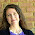Monday, November 3, 2014

How to Teach Algebra with Leaves

Last week, I posted a lesson idea for sharpening students understanding of multiplication and estimation using leaves.  This post is an extension of that same idea, moving it into algebra and allowing the students to get a taste of real world mathematical modeling.

Use Algebra to Analyze Leaves

Start your lesson just like I suggested in the post, How to Teach Multiplication with Leaves.   I would recommend having your students work in groups of 2-4 to accomplish these tasks.  If you are working with more advanced students, you should be able to move quickly to the point of setting up the multiplication equations to find the number of veins in each leaf.

Next, point out that rather than repeating the process for every leaf, it would be faster to make an equation that would work for every leaf, then just plug in the numbers to the equation.  Challenge your students to come up with the equation.

You may want to have them work out some mini-equations first.
#tiniest veins = (tiniest veins/small vein) * #small veins
# small veins = (small veins/medium vein) * # medium veins

The mini-equations can be added together, and the final equation will look like this....
Total = (#Tiniest veins/small vein) *(#small veins) + (#small veins/medium vein) * #medium vein . . .

Of course, this equation can then be simplified.  When the equation is simplified, it becomes apparent that, mathematically, the unique feature of each leaf is the ratio of small veins to larger veins.  Each leaf can be described quickly as a unique set of numbers corresponding to these ratios.  The final step is to describe each leaf as a list of these ratios.

Real World Application

Log into a map program and pull up several maps of cities.  Include your own town, and the nearby cities best known to your students.  Ask your students to compare the highway system to that of the leaves you have just been analyzing.  If all your examples are similar to the leaves, you may want to pull up Washington D.C. as a contrast.  Washington D.C. has a traffic pattern that is radically different from the pattern seen in a tree leaf.  It also has horrible congestion, which illustrates the importance of following natures patterns.

Discuss in class, or assign a short essay on the topic:  "How should the highways and streets of a city be laid out for most efficient traffic flow?"  Ask your students to base their reasoning on the assumption that nature gives us an effective blue print for movement of large number of units, and to use actual numbers in their answers.

I hope this idea is helpful to you in engaging your students in math and making helpful connections with the natural world.  I would love to hear how it works out for you!

Other posts you may enjoy:

1.1.